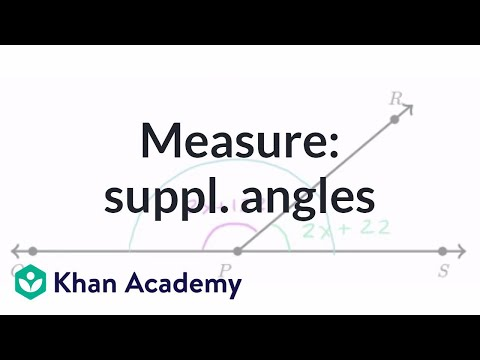Video

# Equation practice with complementary angles (Full video)

Description: Given the algebraic expressions that represent a pair of complementary angles, learn how to form and solve an equation to find an unknown angle. Created by Sal Khan. Then they tell us that the measure of angle LOM is equal to 2x plus 46. So LOM is equal to 2x plus 46. Then they tell us that the measure of angle MON is equal to 3x minus 6.

### Other videos you might be interested in### Complementary & supplementary angles (Full video)